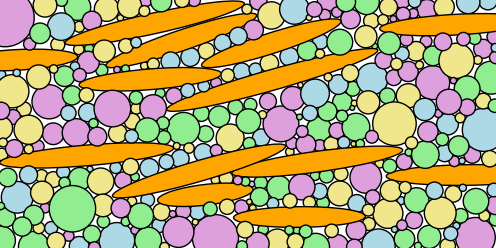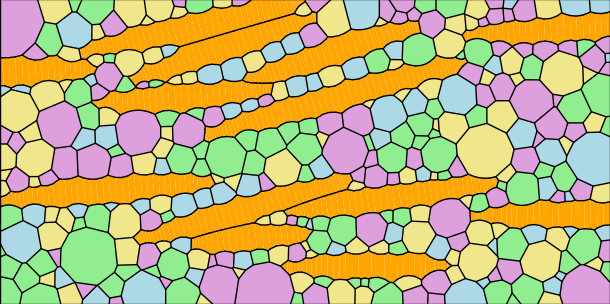# Elliptical Grains¶

## XML Input File¶

The basename for this file is elliptical_grains.xml. The file can be run using this command:

microstructpy --demo=elliptical_grains.xml


The full text of the file is:

<?xml version="0.5" encoding="UTF-8"?>
<input>
<material>
<fraction> 2 </fraction>
<shape> ellipse </shape>
<a>
<dist_type> uniform </dist_type>
<loc> 0.2  </loc>
<scale> 0.35 </scale>
</a>
<b> 0.05 </b>
<angle_deg>
<dist_type> uniform </dist_type>
<loc> 0 </loc>
<scale> 20 </scale>
</angle_deg>
<color> orange </color>
</material>

<material>
<fraction> 1 </fraction>
<shape> circle </shape>
<area>
<dist_type> lognorm </dist_type>
<scale> 0.004 </scale>
<s> 1.0 </s>
</area>
<color> plum </color>
</material>

<material>
<fraction> 1 </fraction>
<shape> circle </shape>
<area>
<dist_type> lognorm </dist_type>
<scale> 0.004 </scale>
<s> 1.0 </s>
</area>
<color> lightblue </color>
</material>

<material>
<fraction> 1 </fraction>
<shape> circle </shape>
<area>
<dist_type> lognorm </dist_type>
<scale> 0.004 </scale>
<s> 1.0 </s>
</area>
<color> lightgreen </color>
</material>

<material>
<fraction> 1 </fraction>
<shape> circle </shape>
<area>
<dist_type> lognorm </dist_type>
<scale> 0.004 </scale>
<s> 1.0 </s>
</area>
<color> khaki </color>
</material>

<domain>
<shape> rectangle </shape>
<side_lengths> (2.4, 1.2) </side_lengths>
</domain>

<settings>
<verbose> True </verbose>
<mesh_min_angle> 20 </mesh_min_angle>
<mesh_max_edge_length> 0.01 </mesh_max_edge_length>
<mesh_max_volume> 0.004 </mesh_max_volume>

<directory> elliptical_grains </directory>
<plot_axes> False </plot_axes>

<seeds_kwargs>
<linewidth> 1.0 </linewidth>
</seeds_kwargs>

<poly_kwargs>
<linewidth> 1.0 </linewidth>
</poly_kwargs>

<tri_kwargs>
<linewidth> 0.1 </linewidth>
</tri_kwargs>
</settings>
</input>


## Materials¶

There are five materials, represented in equal proportions. The first material consists of ellipses and the semi-major axes are uniformly distributed, $$A \sim U(0.20, 0.75)$$. The semi-minor axes are fixed at 0.05, meaning the aspect ratio of these seeds are 4-15. The orientation angles of the ellipses are uniform random in distribution from 0 to 20 degrees counterclockwise from the +x axis.

The remaining four materials are all the same, with lognormal grain area distributions. The only difference among these materials is the color, which was done for visual effect.

## Domain Geometry¶

The domain of the microstructure is a rectangle with side lengths 2.4 in the x-direction and 1.2 in the y-direction. The domain is centered on the origin, though the position of the domain is not relevant considering that the plot axes are switched off.

## Settings¶

The aspect ratio of elements in the triangular mesh is controlled by setting the minimum interior angle for the elements at 20 degrees, the maximum element volume to 0.001, and the maximum edge length at grain boundaries to 0.01.

The function will output only plots of the microstructure process (no text files), and those plots are saved as PNGs. They are saved in a folder named elliptical_grains, in the current directory (i.e ./elliptical_grains).

The axes are turned off in these plots, creating PNG files with minimal whitespace.

Finally, the linewiths in the seeds plot, polygonal mesh plot, and the triangular mesh plot are 0.5, 0.5, 0.1 respectively.

## Output Files¶

The three plots that this file generates are the seeding, the polygon mesh, and the triangular mesh. These three plots are shown in Fig. 4 - Fig. 6.Fig. 4 Elliptical grain example - seed geometries.¶Fig. 5 Elliptical grain example - polygonal mesh.¶Fig. 6 Elliptical grain example - triangular mesh.¶